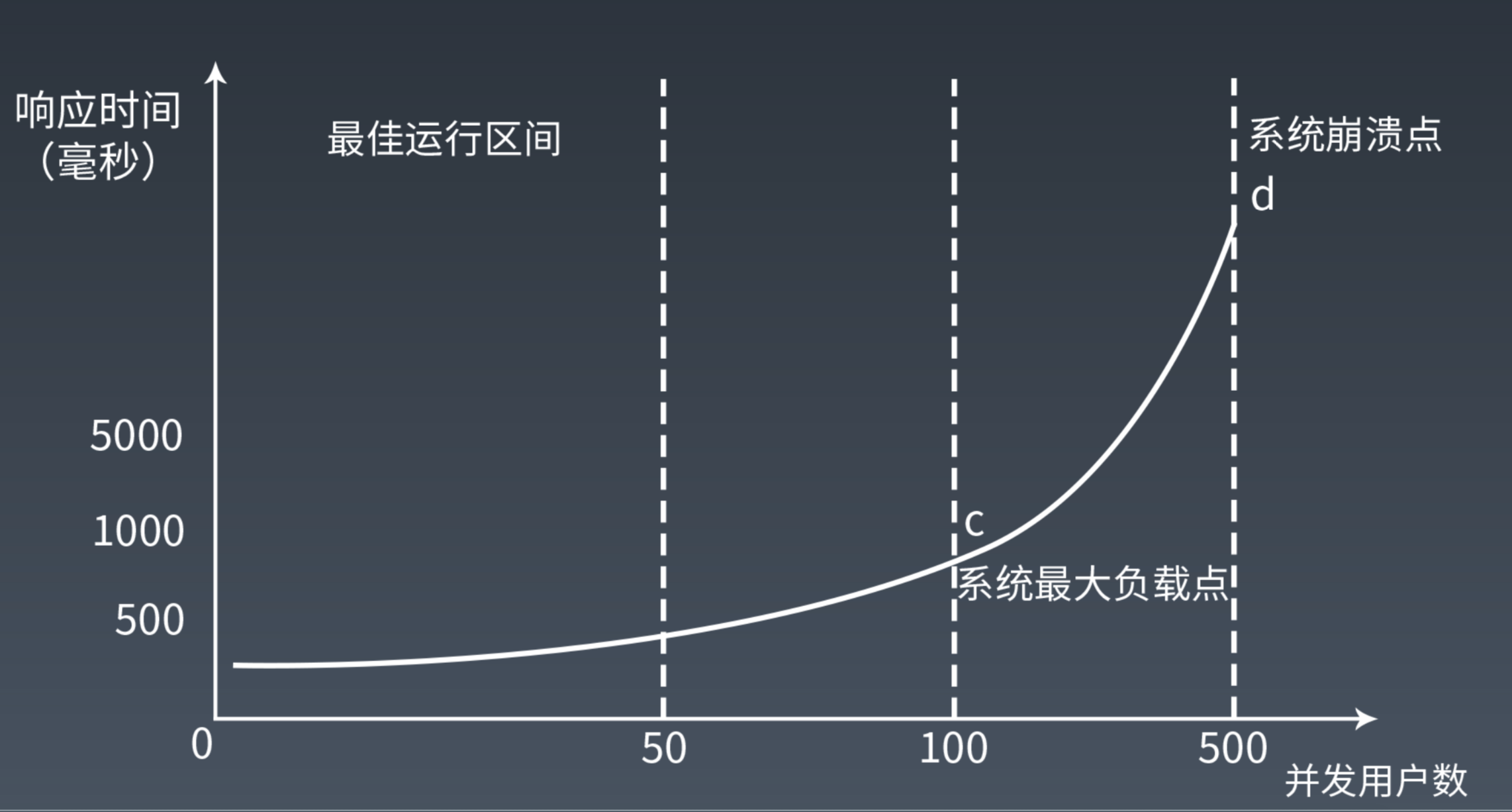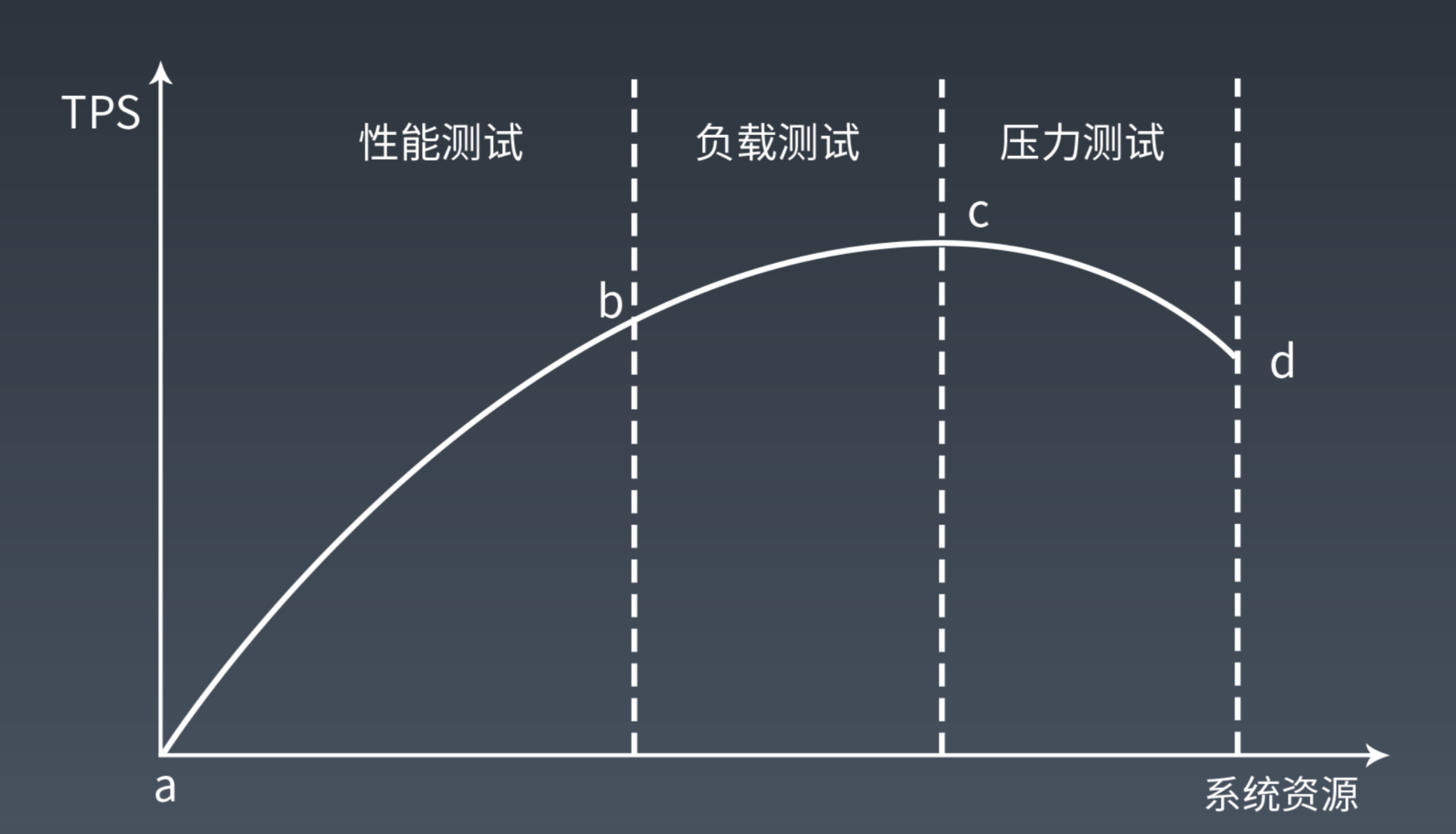# 架构师训练营 性能压测命题作业Cloud.

• 性能压测的时候，随着并发量的增加，系统响应时间和吞吐量如何变化？为什么？

• 系统响应时间在并发上升初期会缓慢上升，而达到临界点后会急剧上升• 吞吐量在早期会随着并发升高很快上升，而随着系统响应时间在临界点的急剧上升，吞吐量会开始急剧下降• 用你熟悉的编程语言写一个 web 性能压测工具，输入参数：URL，请求总次数，并发数。输出参数：平均响应时间，95% 响应时间。用这个测试工具以 10 并发、100 次请求压测 www.baidu.com。

public class Press {        private String url;    private int concurrent;    private int count;    private ExecutorService executorService;    private Statistics statistics;    public Press(String url, int concurrent, int count) {        this.url = url;        this.concurrent = concurrent;        this.count = count;        this.statistics = new Statistics();        this.executorService = new ThreadPoolExecutor(concurrent, concurrent, 400, TimeUnit.SECONDS,                                                      new LinkedBlockingDeque<>(concurrent));    }    public Statistics execute() throws InterruptedException {        CountDownLatch latch = new CountDownLatch(10);        for (int i = 0; i < concurrent; i++) {            this.executorService.execute(() -> {                try {                    new Request(url, statistics).request(count);                } finally {                    latch.countDown();                }            });        }        latch.await();        return statistics;    }    public static void main(String[] args) throws InterruptedException {        String url = "http://www.baidu.com";        int concurrent = 10;        int count = 300;        Statistics statistics = new Press(url, concurrent, count).execute();        System.out.println("平均执行耗时：" + statistics.avgCost());        System.out.println("95%耗时在：" + statistics.cost95());    }}

public class Request {    private String url;    private Statistics statistics;    private RestTemplate restTemplate;    public Request(String url, Statistics statistics) {        this.url = url;        this.statistics = statistics;        this.restTemplate = new RestTemplate();    }    public void request(int count) {        for (int i = 0; i < count; i++) {            long start = System.currentTimeMillis();            restTemplate.exchange(url, HttpMethod.GET, null, String.class);            long end = System.currentTimeMillis();            statistics.add(end - start);        }    }    public static void main(String[] args) {        RestTemplate restTemplate = new RestTemplate();        String url = "http://www.baidu.com";        ResponseEntity<String> str = restTemplate.exchange(url, HttpMethod.GET, null, String.class);        System.out.println(str.getBody());    }}

public class Statistics {        private AtomicInteger count;    private List<Long> requestCost;    public Statistics() {        this.count = new AtomicInteger(0);        this.requestCost = new CopyOnWriteArrayList<>();    }    public void add(long cost) {        count.incrementAndGet();        requestCost.add(cost);    }    public BigDecimal avgCost() {        long total = requestCost.stream().mapToLong(o -> o).sum();        return BigDecimal.valueOf(total).divide(BigDecimal.valueOf(count.get()), 2, BigDecimal.ROUND_UP);    }    public long cost95() {        Collections.sort(requestCost);        int index = (int)(count.get() * 0.95);        int offset = count.get() - index;        return requestCost.get(offset);    }}

平均执行耗时：39.7395%耗时在：30## 评论